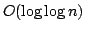Statistica Sinica 16(2006), 1335-1365

STRONG LIMIT THEOREMS ON MODEL SELECTION IN

GENERALIZED LINEAR REGRESSION WITH

BINOMIAL RESPONSES

Guoqi Qian and Yuehua Wu

University of Melbourne and York University

Abstract: We prove a law of iterated logarithm for the maximum likelihood estimator of the parameters in a generalized linear regression model with binomial response. This result is then used to derive an asymptotic bound for the difference between the maximum log-likelihood function and the true log-likelihood. It is further used to establish the strong consistency of some penalized likelihood based model selection criteria. We have shown that, under some general conditions, a model selection criterion will select the simplest correct model almost surely if the penalty term is an increasing function of the model dimension and has an order betweenand. Cases involving the commonly used link functions are discussed for illustration of the results.

Key words and phrases: Generalized linear models, law of the iterated logarithm, maximum likelihood estimator, model selection, strong consistency.